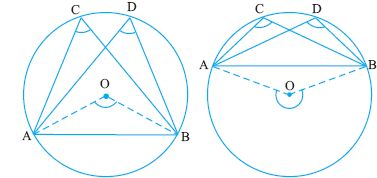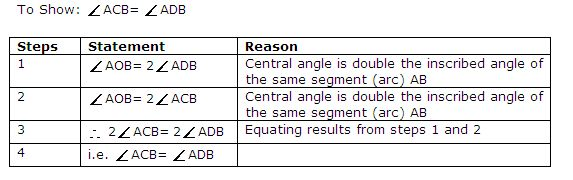Name: ___________________Date:___________________

 Email us to get an instant 20% discount on highly effective K-12 Math & English kwizNET Programs!

### High School Mathematics - 28.4 Theorems - Circles

 Theorem: Angle is a semi circle is a right angle.Given : Angle AOB is in semi circle. To prove: Angle ACB = 90o Proof: Angle ACB = 1/2 angle AOB (angle subtended at the centre is twice angle at circumference) Angle AOB = 180o (straight line) Hence ACB = 90o Theorem: Angles in the same segment of a circle are equal.Directions: Solve the following
 Q 1: Find x.35 degrees140 degrees70 degrees Question 2: This question is available to subscribers only!